Question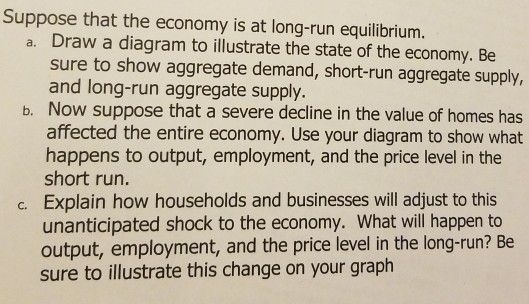(a)

In following graph, initial long-run equilibrium is at point A where AD0 (aggregate demand), LRAS0 (long-run aggregate supply) and SRAS0 (short-run aggregate supply) curves intersect with long-run equilibrium price level P0 and long-run equilibrium real GDP (which is equal to potential GDP) Y0.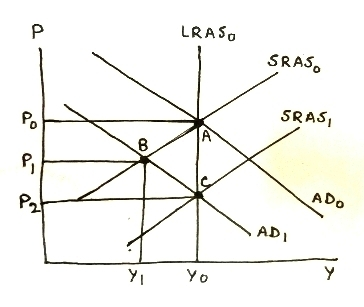(b)

Decline in value of home will decrease consumer wealth, so they will reduce consumption. A decrease in consumption will decrease aggregate demand. AD curve will shift to left, reducing both price level and real GDP, giving rise to a recessionary gap in short run. Lower real GDP will increase unemployment rate.

In above graph, when aggregate demand falls, AD curve will shift leftward from AD0 to AD1, intersecting SRAS0 at point B with lower price level P1 and lower real output Y1, with short run recessionary gap of (Y0 - Y1).

(c)

In the long run, lower price level will reduce wages and prices of inputs, lowering production costs. Firms will increase output, increasing aggregate supply. SRAS shifts rightward, intersecting new AD curve at further lower price level but restoring real GDP to potential GDP level, wiping out the short-run recessionary gap. Unemployment rate will restore at natural (full-employment) rate.

In above graph, in long run, SRAS0 shifts right to SRAS1, intersecting AD1 at point C with further lower price level P2 and restoring real GDP to potential GDP level Y0, removing the short-run recessionary gap.

#### Earn Coins

Coins can be redeemed for fabulous gifts.

Similar Homework Help Questions
• ### Question 1) Suppose the economy is operating at both short-run and long-run equilibrium. Suppose consumers’ wealth incre...

Question 1) Suppose the economy is operating at both short-run and long-run equilibrium. Suppose consumers’ wealth increases, and they begin spending more on Tide Pod laundry detergent for entertainment purposes. Draw the graphs and shifts for the following questions and provide the specific mechanisms and channels. a) What happens in the short run to both the aggregate price level and aggregate output when this shock occurs? b) What will happen in the long run to both aggregate price level and...

• ### IV. Suppose an economy is in long run equilibrium. (a) Use the model of aggregate demand...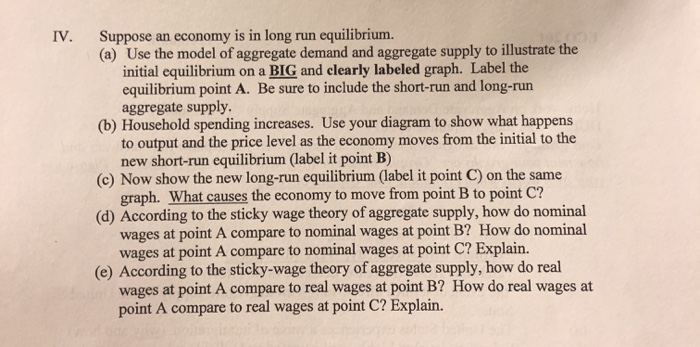IV. Suppose an economy is in long run equilibrium. (a) Use the model of aggregate demand and aggregate supply to illustrate the initial equilibrium on a BIG and clearly labeled graph. Label the equilibrium point A. Be sure to include the short-run and long-run aggregate supply. (b) Household spending increases. Use your diagram to show what happens to output and the price level as the economy moves from the initial to the new short-run equilibrium (label it point B) (c)...

• ### QUESTION 5-1 Chapter 14 Suppose economy is in long run equilibrium. [Only one diagram is required...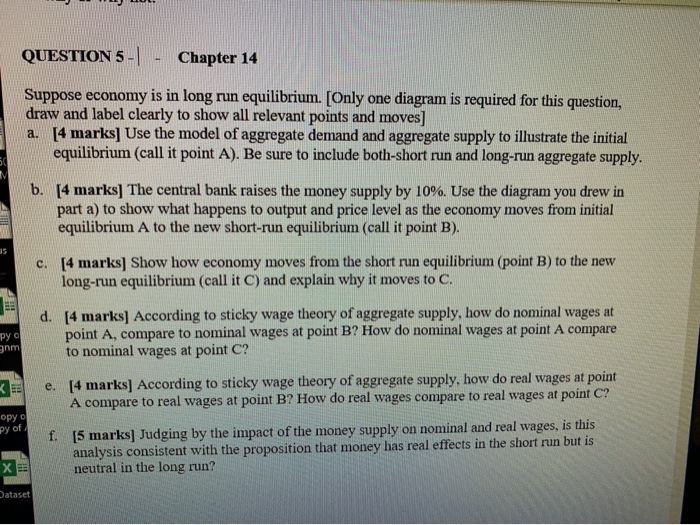QUESTION 5-1 Chapter 14 Suppose economy is in long run equilibrium. [Only one diagram is required for this question, draw and label clearly to show all relevant points and moves] a. [4 marks] Use the model of aggregate demand and aggregate supply to illustrate the initial equilibrium (call it point A). Be sure to include both-short run and long-run aggregate supply. b. [4 marks] The central bank raises the money supply by 10%. Use the diagram you drew in part...

• ### The following graph shows the economy in long-run equilibrium atthe expected price level of 120...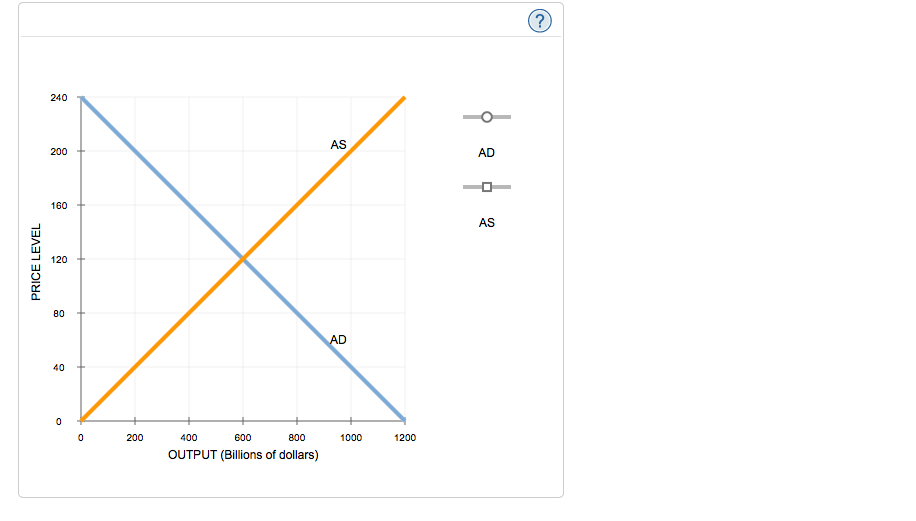The following graph shows the economy in long-run equilibrium at the expected price level of 120 and the natural level of output of \$600 billion. Suppose a sudden and severe contraction in the housing market reduces the value of homes and causes consumers to spend less.Shift the short-run aggregate supply (AS) curve or the aggregate demand (AD) curve to show the short-run impact of the housing market slump.In the short run, the decrease in consumption spending associated with the housing...

• ### • draw an aggregate demand and aggregate supply diagram to illustrate your answer • show the...

• draw an aggregate demand and aggregate supply diagram to illustrate your answer • show the change in aggregate demand and/or aggregate supply • describe the change(s) you have shown • explain why the adjustments you have described occur. 1. Suppose that there is an expansion of private consumption due to increased optimism about future growth prospects for the economy. (i) Illustrate and explain the effect of this shock in the short-run. (ii) What is the long-run effect likely to...

• ### The graphs illustrate an initial equilibrium for the economy. Suppose that the stock market broadly decreases....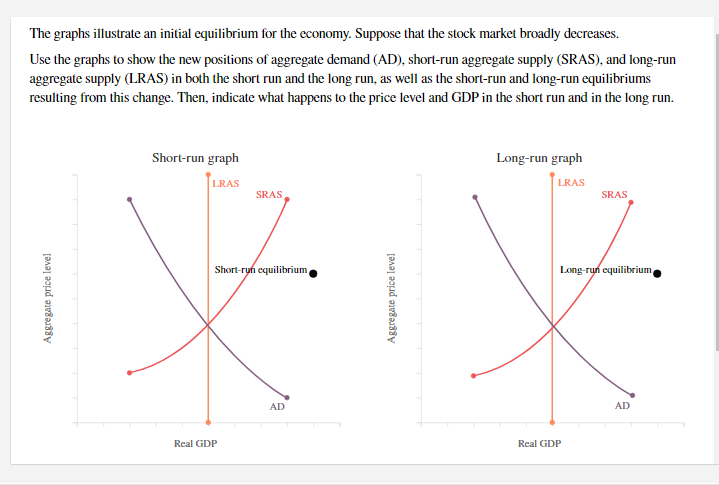The graphs illustrate an initial equilibrium for the economy. Suppose that the stock market broadly decreases. Use the graphs to show the new positions of aggregate demand (AD), short-run aggregate supply (SRAS), and long-run aggregate supply (LRAS) in both the short run and the long run, as well as the short-run and long-run equilibriums resulting from this change. Then, indicate what happens to the price level and GDP in the short run and in the long run. Short-run graph Long-run...

• ### The graphs illustrate an initial equilibrium for the economy. Suppose that oil prices temporarily decrease Use...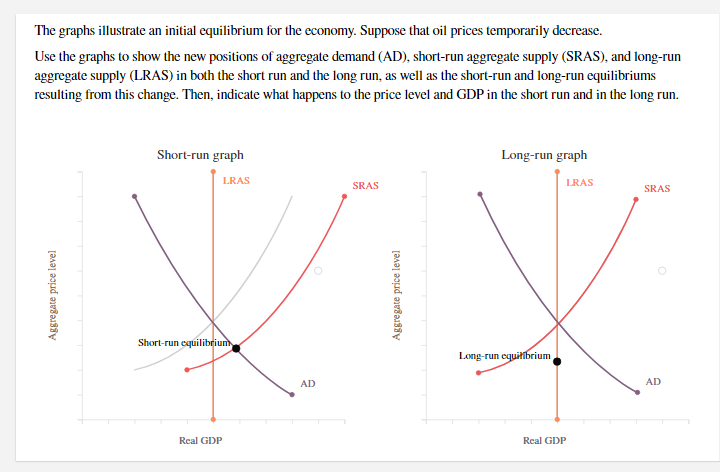The graphs illustrate an initial equilibrium for the economy. Suppose that oil prices temporarily decrease Use the graphs to show the new positions of aggregate demand (AD), short-run aggregate supply (SRAS), and long-run aggregate supply (LRAS) in both the short run and the long run, as well as the short-run and long-run equilibriums resulting from this change. Then, indicate what happens to the price level and GDP in the short run and in the long run. Short-run graph Long-run graph...

• ### Economics chart The following graph shows the economy in long-run equilibrium at the price level of...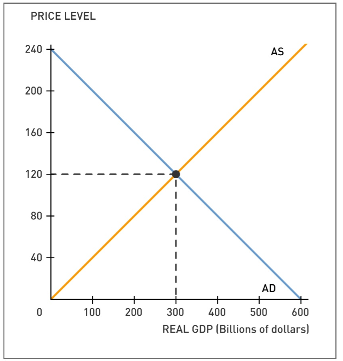Economics chart The following graph shows the economy in long-run equilibrium at the price level of 120 and potential output of \$300 billion. Suppose several foreign economies experience severe recessions, causing foreign purchases of domestic goods and services to decline sharply. Shift the short-run aggregate supply (AS) curve or the aggregate demand (AD) curve to show the short-run impact of the economic turmoil abroad. Tool tip: Click and drag one or both of the curves. Curves will snap into position, so if...

• ### 2. Suppose the economy is in long-run equilibrium, with real GDP at \$19 trillion and the unemployment rate at 5%. Now assume that the central bank unexpectedly decreases money supply by 6%. a) Illust...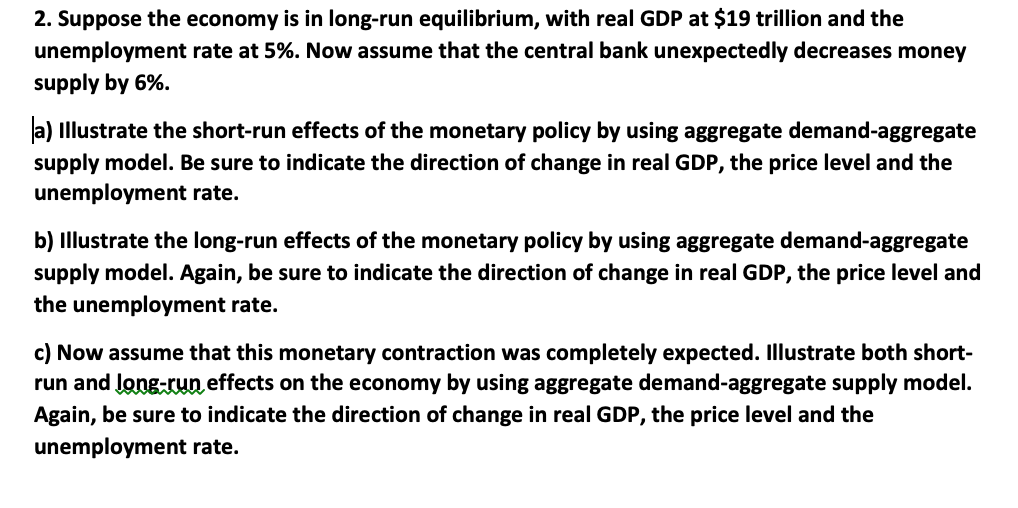2. Suppose the economy is in long-run equilibrium, with real GDP at \$19 trillion and the unemployment rate at 5%. Now assume that the central bank unexpectedly decreases money supply by 6%. a) Illustrate the short-run effects of the monetary policy by using aggregate demand-aggregate supply model. Be sure to indicate the direction of change in real GDP, the price level and the unemployment rate. b) Illustrate the long-run effects of the monetary policy by using aggregate demand-aggregate supply model....

• ### Beginning with long-run equilibrium, use the aggregate demand and aggregate supply model to illustrate what happens...

Beginning with long-run equilibrium, use the aggregate demand and aggregate supply model to illustrate what happens in the short run when the economy suffers a negative supply shock. (10 points)